# Euros

Michal, Peter, John, and Lenka got together 2,400 euros. They share an amount in ratio 2:6:4:3. How many got each of them?

m =  320
p =  960
j =  640
l =  480

### Step-by-step explanation: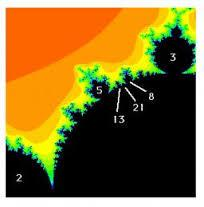Did you find an error or inaccuracy? Feel free to write us. Thank you!Tips to related online calculators
Check out our ratio calculator.

## Related math problems and questions:

• Composite ratioJakub, Aneta, and Lenka divided 1342 USD in the ratio 5/2: 3/10: 1/4. How much did Lenka take?
• Money split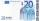Anton and Ferko got 2,500 euros together. The amount was split in a 1:4 ratio. How much did each get?
• TogetherThe three friends divided the balls in a ratio of 6: 5: 4. Some two of them got a total of 126 balls. How many balls were there together?
• Moneys in triple ratioMilan, John and Lili have a total 344 euros. Their amounts are in the ratio 1:2:5. Determine how much each of them has?
• Revenue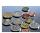Mr. Robert and Mr. Norbert get together € 1400. The revenue split in the ratio of 4:3, depending upon each work. How many got each of them?
• ErikThree friends Peter, Erik and Milan shared 420 euros in a ratio of 1: 2: 3. How many euros did Erik get?
• Three 43Three brothers inherited a cash amount of 62,000, and they divided it among themselves in the ratio of 5:4:1. How much more is the largest share than the smallest share?
• A sumA sum of money is shared between Peter, John and Henry in the ratio 2:3:5. a) express Henry's share as fraction of John's share. b) what fraction of the whole sum of money is John's share?
• Two discounts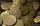The MP4 player cost 960 CZK, the price was twice gradually reduced in the ratio 4: 3: 3/2. How much did the player cost after the second discount?
• Three friendsJohn, Peter, and Pablo each carried a 24 liters bucket full of water down the hill. After they reached the bottom, John's bucket was only 3/4 full, Peter's bucket was 2/3 full, and Pablo's was 1/6 full. How much liters of water did they spill altogether o
• AgesJohn, Teresa, Daniel and Paul have summary 56 years. Their ages are in a ratio of 1:2:5:6. Determine how many years have each of them.
• Change the numbers in the ratio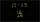Change the numbers 29, 38, and 43 in a 3: 4 ratio.
• Division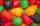Three siblings Helena, Oliver and George split the bag with candies on merit in the ratio 6:1:4. How many candies should each get if in bag were 88?
• Troops - soldiers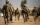Food stocks will last 20 soldiers of the 4-day training. How many days they could extend training if four soldiers become sick?
• Three workersThree workers got paid for work. First earn € 50, the second € 100 and third € 60. For further work, we should receive together € 735. How do you divide that amount to be distributed in the same proportion as the first job?
• Dividing moneyMilan divided 360 euros in the ratio 4: 5, Erik in the ratio 500: 625. How many euros did the individual parts of Milan and how many Erik?
• Unknown amount of money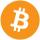Damian and Denis split an unknown amount in the ratio of 5:4. Damian got six euros more than Denis. Calculate an unknown amount. Determine how much money got Damian and how Denis.